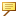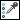Surfacing > Facet Modeling > Point Handling > Sample > To Delete Points by Sampling
To Delete Points by Sampling
1. Select a point set area to sample.If you do not select an area, points are deleted by sampling from the entire point set.
2. Click Points >Sample. The Sample dialog box opens.
3. Select the method of sampling.
Random Sample—Randomly removes the specified percentage of points from the point set.
In the Sample Percentage box, type a value for a percentage of the original points that you want to keep.
Uniform Sample—Removes points from the point set uniformly. This is done by dividing the point set's model space into equal-sized cubes and deleting all but one point from each cube.
In the Spacing box, type a value for the edge length of the cube. You can also click the selection arrow and select two points from the point set to determine the size of the cube.A large cube size means that fewer points are retained from the point set whereas a small cube size retains more points.
Sample by Curvature—Removes points from the point set  depending on the surface curvature at that point.
More points in the areas of high curvature are retained to maintain the accuracy in that area of the surface. Fewer points in the regions with less curvature or flat regions are retained as these regions require less detail.
In the Sample Percentage box, type a value for a percentage of the original points that you want to keep.
4. Click OK.